Maths-
General
Easy

Question

# Calculate the area of equilateral triangle whose height is 25 cmHint:

## The correct answer is: 1.73

### It is given that height, h = 25 cmLet AB = xWe know that all angles are equal to 60°.Sin A =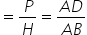Sin 60° =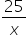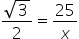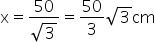Since all sides are equal BC =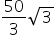We know that Area of triangle =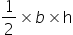=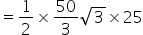= 208.33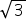= 360.84 cm2                       ( ∴= 1.73 )NOTE – We can use Pythagoras theorem too. Since perpendicular of an equilateral triangle bisects the side, implies that BD =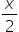. So, in triangle ABDAB2 = AD2 + BD2Find x and then use Area of triangle =#### With Turito Foundation.#### Get an Expert Advice From Turito.